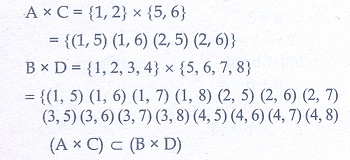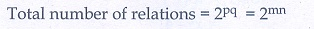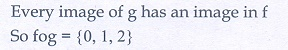Home | | Maths 10th Std | Multiple choice questions

# Multiple choice questions

Mathematics: Relation and Function: Multiple choice questions with answers / choose the correct answer with answers - Maths Book back 1 mark questions and answers with solution for Exercise Problems

Multiple choice questions

1. If  n(A× B) = 6 and = {1, 3} then n(B) is

(1) 1

(2) 2

(3) 3

(4) 6

Solution2. A = {a,bp},  = {2, 3}, C  = {p,q,r,s} then n[( )× B] is

(1) 8

(2) 20

(3) 12

(4) 16

Solution3. If  = {1, 2}, = {1, 2, 3, 4}, C  = {5, 6} and = {5, 6, 7, 8} then state which of the following statement is true.

(1) (A× (× D)

(2) (× D (A×)

(3) (A× B (A× D)

(4) (× A (× A)

Solution4. If there are 1024 relations from a set = {1, 2, 3, 4, 5} to a set B, then the number of elements in is

(1) 3

(2) 2

(3) 4

(4) 8

Solution5. The range of the relation = {(xx2 ) | x is a prime number less than 13} is

(1) {2,3,5,7}

(2) {2,3,5,7,11}

(3) {4,9,25,49,121}

(4) {1,4,9,25,49,121}

Solution6. If the ordered pairs (+ 2, 4) and (5, 2b) are equal then (a,b) is

(1) (2, –2)

(2) (5,1)

(3) (2,3)

(4) (3, –2)

Solution7. Let n(A) = m  and n(B) = then the total number of non-empty relations that can be defined from to is

(1) Mn

(2) Nm

(3) 2mn – 1

(4) 2mn

Solution8. If {(a, 8),(6,b)} represents an identity function, then the value of and are respectively

(1) (8,6)

(2) (8,8)

(3) (6,8)

(3) (6,6)

9. Let = {1, 2, 3, 4} and = {4, 8, 9,10}. function f→ given by f  = {(1, 4),(2, 8),(3, 9),(4,10)} is a

(1) Many-one function

(2) Identity function

(3) One-to-one function

(4) Into function

10. If (x) = 2x2 and g(x) = 1/3x then o isAns: (3)

Solution11. If f:  B is a bijective function and if n(B) = 7 , then n(A) is equal to

(1) 7

(2) 49

(3) 1

(4) 4

12. Let and be two functions given by

f  = {(0,1),(2, 0),(3,−4),(4, 2),(5, 7)}

= {(0, 2),(1, 0),(2, 4),(−4, 2),(7, 0)}

then the range of o is

(1) {0,2,3,4,5}

(2) {–4,1,0,2,7}

(3) {1,2,3,4,5}

(4) {0,1,2}

Solution13. Let (x) =then

(1) (xy) = (x).(y)

(2) (xy) ≥  (x).(y)

(3) (xy) ≤ (x).(y)

(4) None of these

Solution14. If  = {(1,1),(2, 3),(3, 5),(4, 7)} is a function given by  g(x) = αx β  then the values of and are

(1) (–1,2) )

(2) (2, –1)

(3) (–1, –2)

(4) (1,2)

Solution15. (x) = (+ 1)3 − (− 1)3 represents a function which is

(1) linear

(2) cubic

(3) reciprocal

Solution